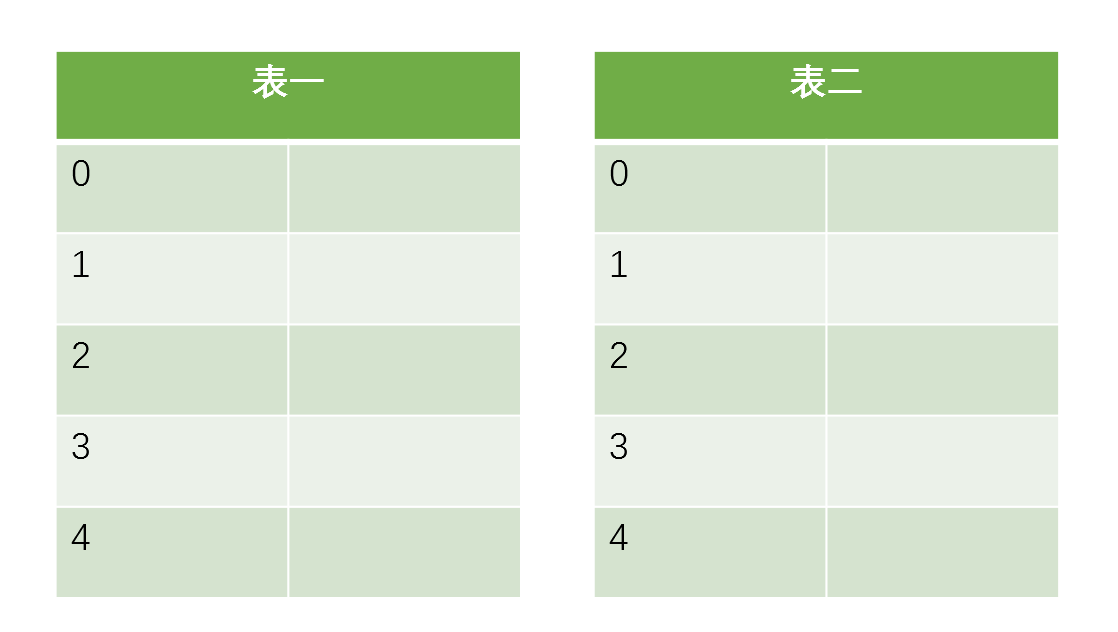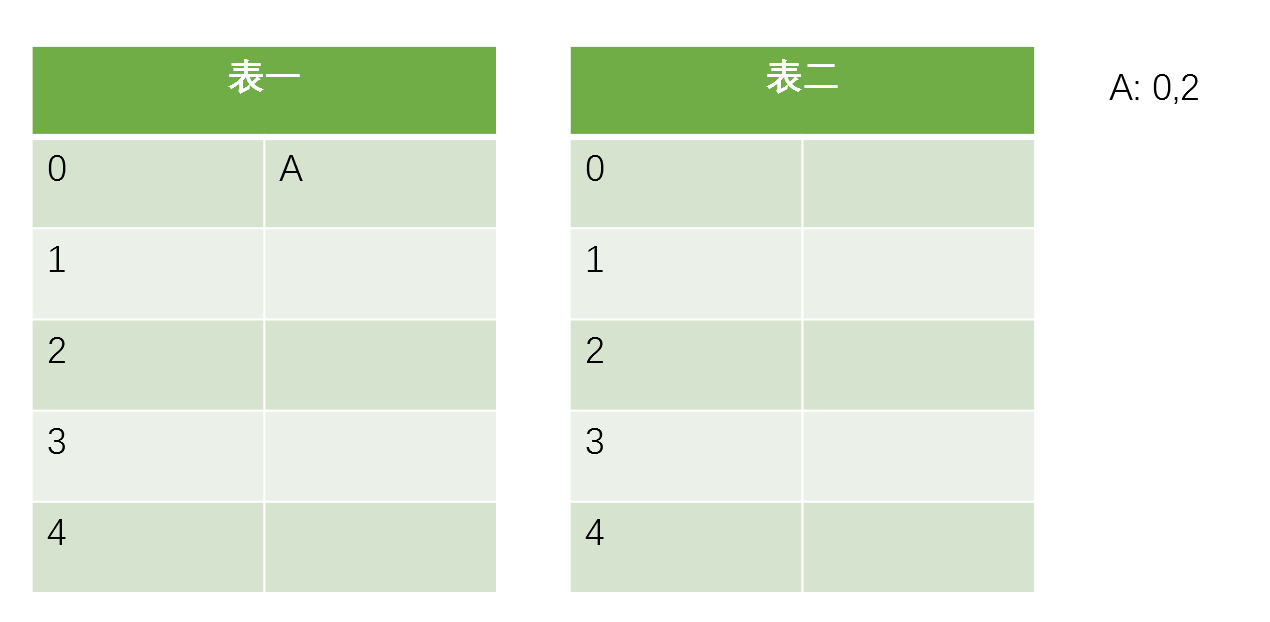没有冲突，顺利搞定，接着插入D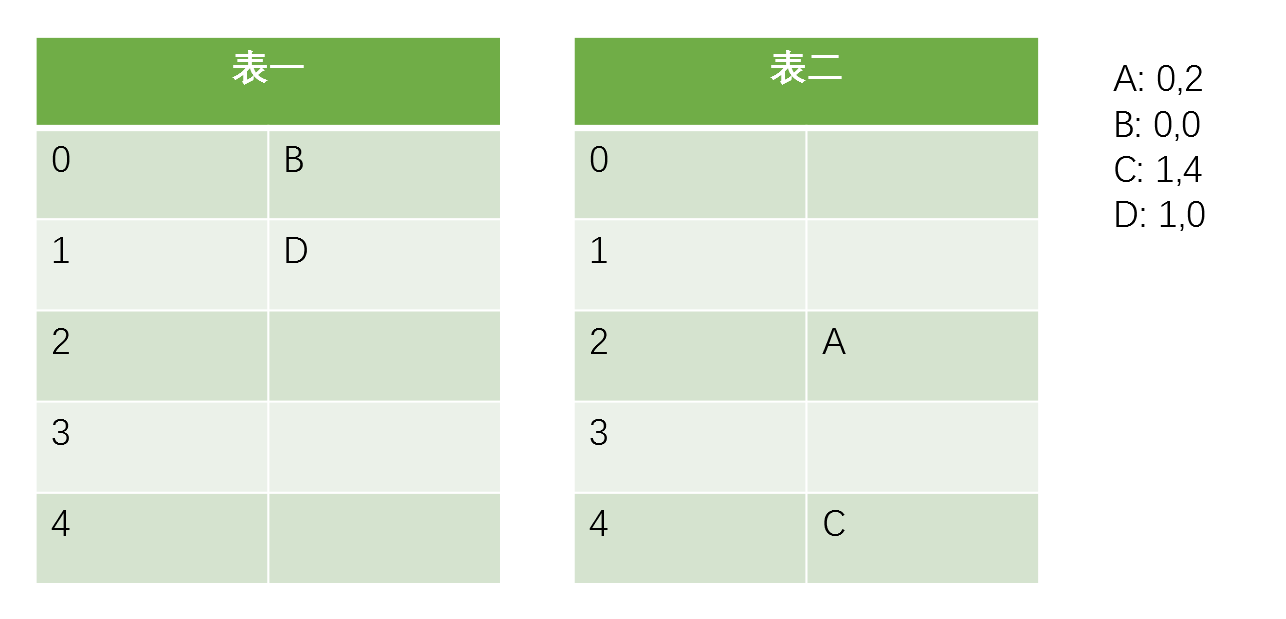D成功的把C踢走了，其实看到这里读者应该在猜想，会不会有一种情况，即被踢走的数据的另一个备选位置也被占用了，这样怎么办？答案是继续踢，一个踢一个，直到大家都找到自己合适的归宿为止。那么如果发现出现了循环怎么办？答案是GG，这代表布谷散列表走到了极限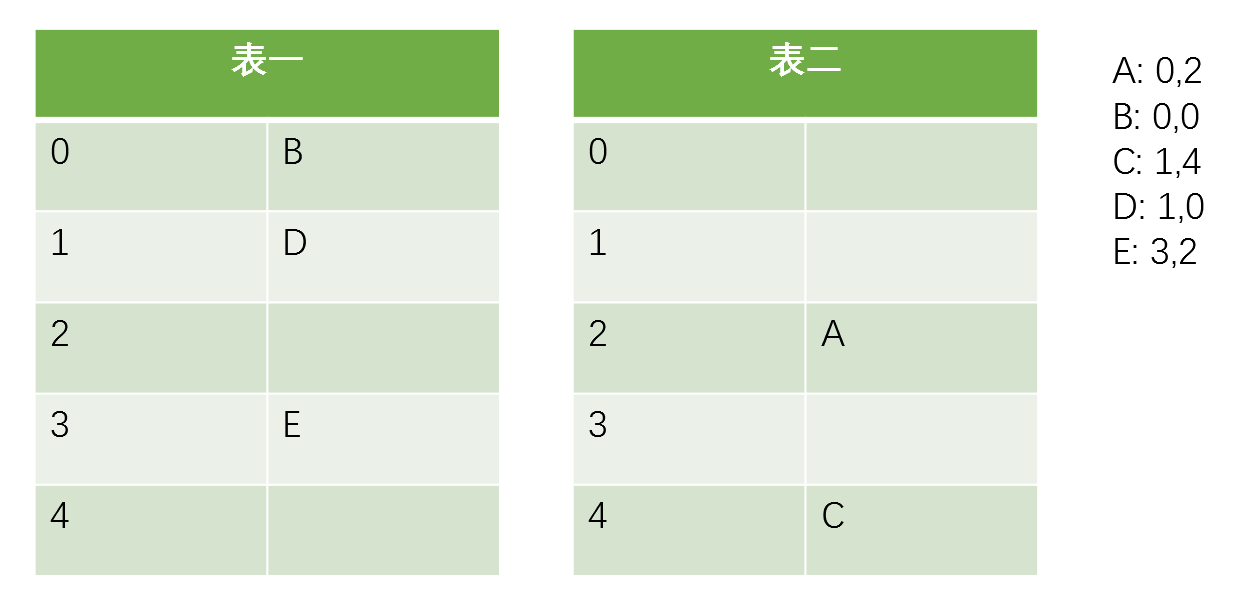插入E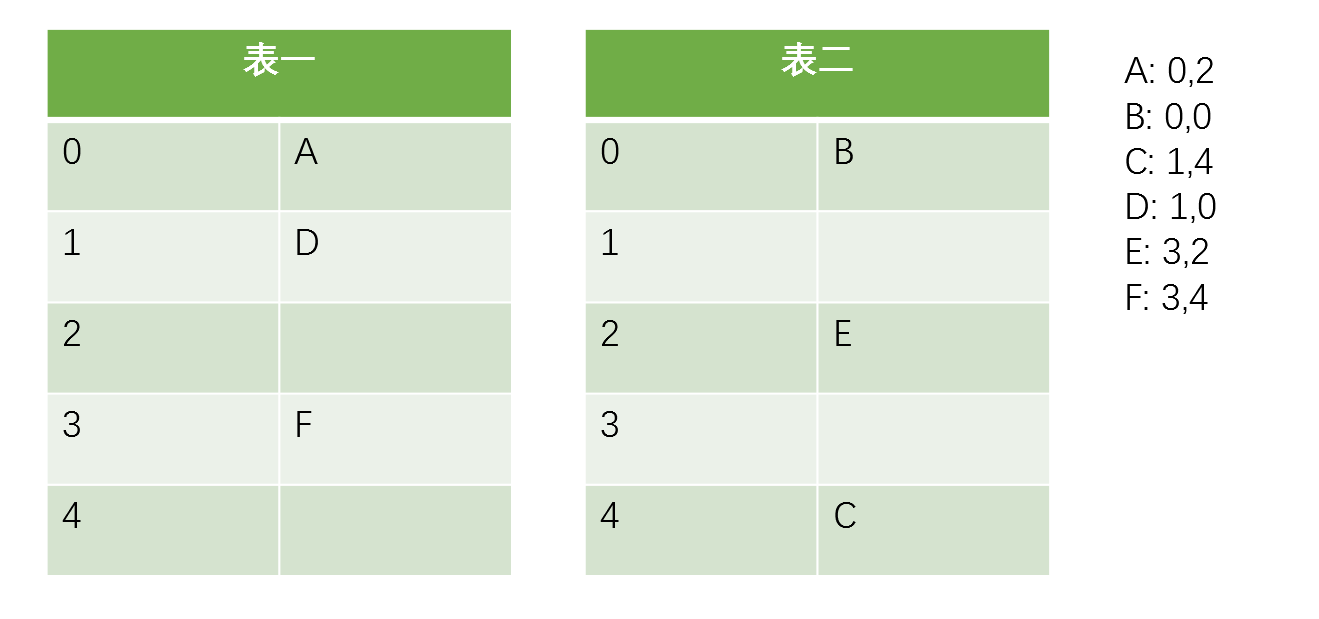这里就发生了多次替换的情况，F代替了E，E代替了A，A代替了B，B找到了空余的位子

  1 2 3 4 5 6 7 8 9 10 11 12 13 14 15 16 17 18  Algorithm 1: Insert(x) f = fingerprint(x) i1 = hash(x) i2 = i1 xor hash(f) if bucket[i1] or bucket[i2] has an empty entry then //只要有空位就先插入空位里 add f to that bucket return Done i = randomly pick i1 or i2 for n=0;n

 1 2 3 4 5 6 7  Algorithm 2: Lookup(x) f = fingerprint(x) i1 = hash(x) i2 = i1 xor hash(f) if bucket[i1] or bucket[i2] has f then return True return False 

 1 2 3 4 5 6 7 8  Algorithm 3: Delete(x) f = fingerprint(x) i1 = hash(x) i2 = i1 xor hash(f) if bucket[i1] or bucket[i2] has f then remove a copy of f from this bucket return False 

$f \ge log_2^{2b/\epsilon} = log_2^{1/\epsilon} + log_2^{2b}$

$C \le (log_2^{1/\epsilon} + 3) / \alpha$